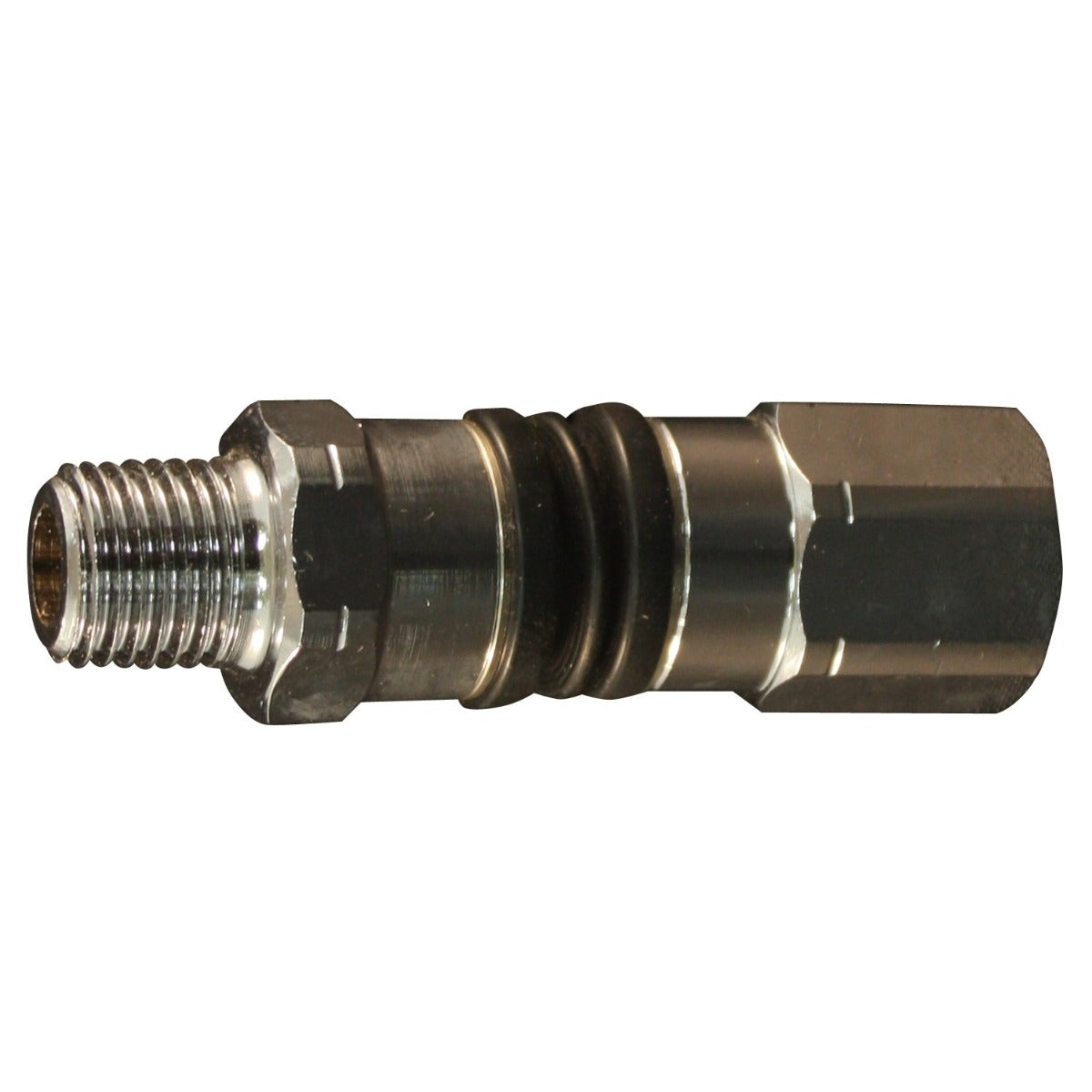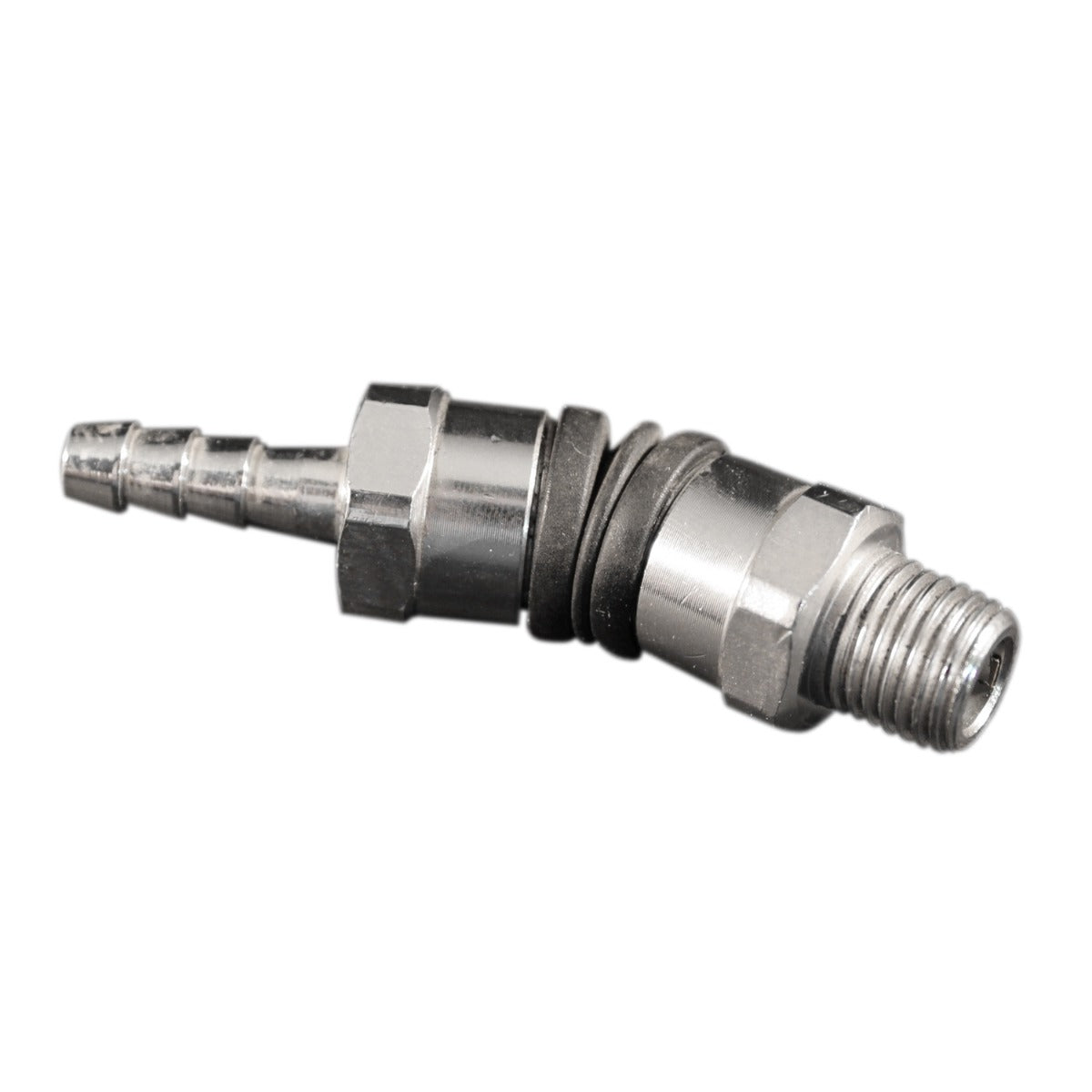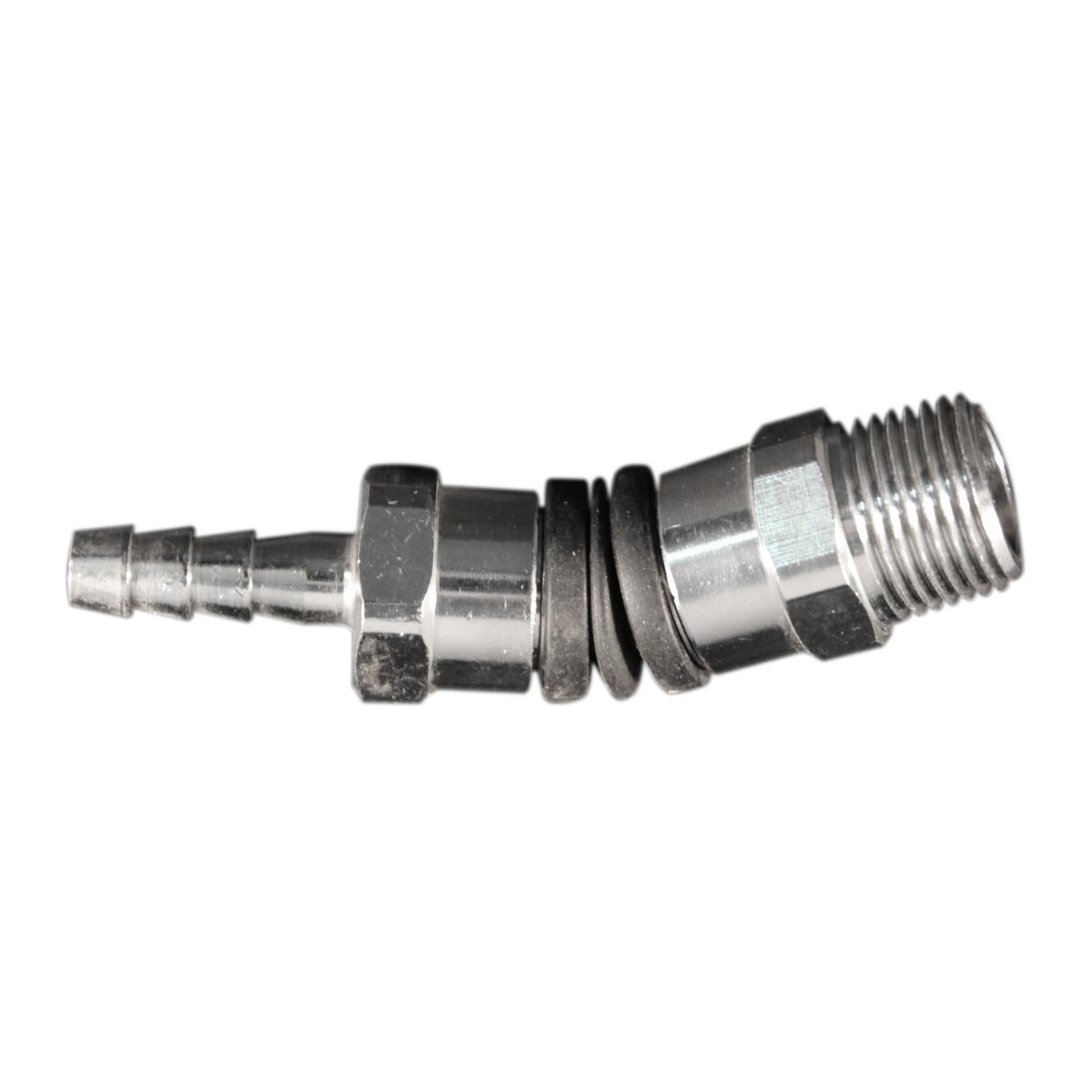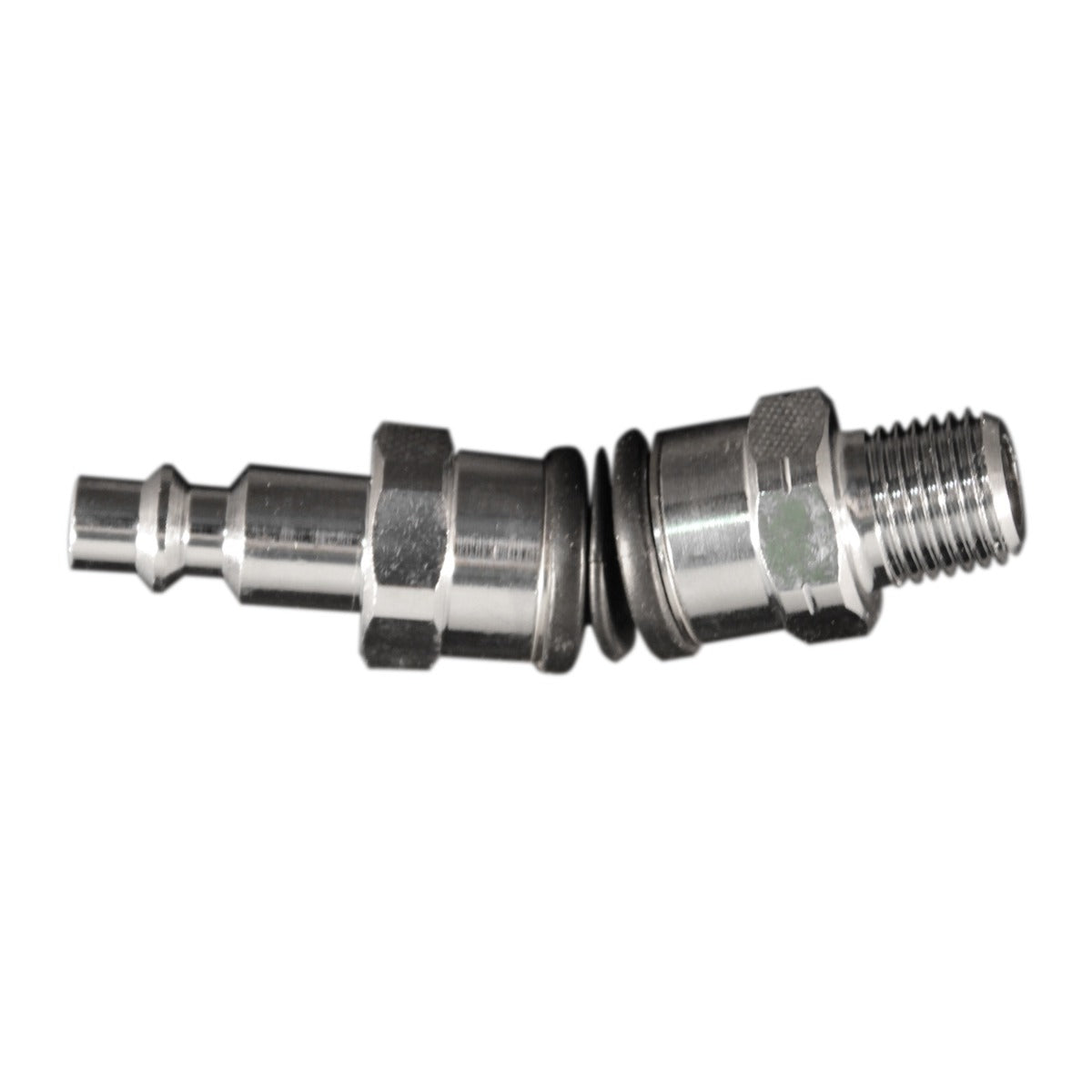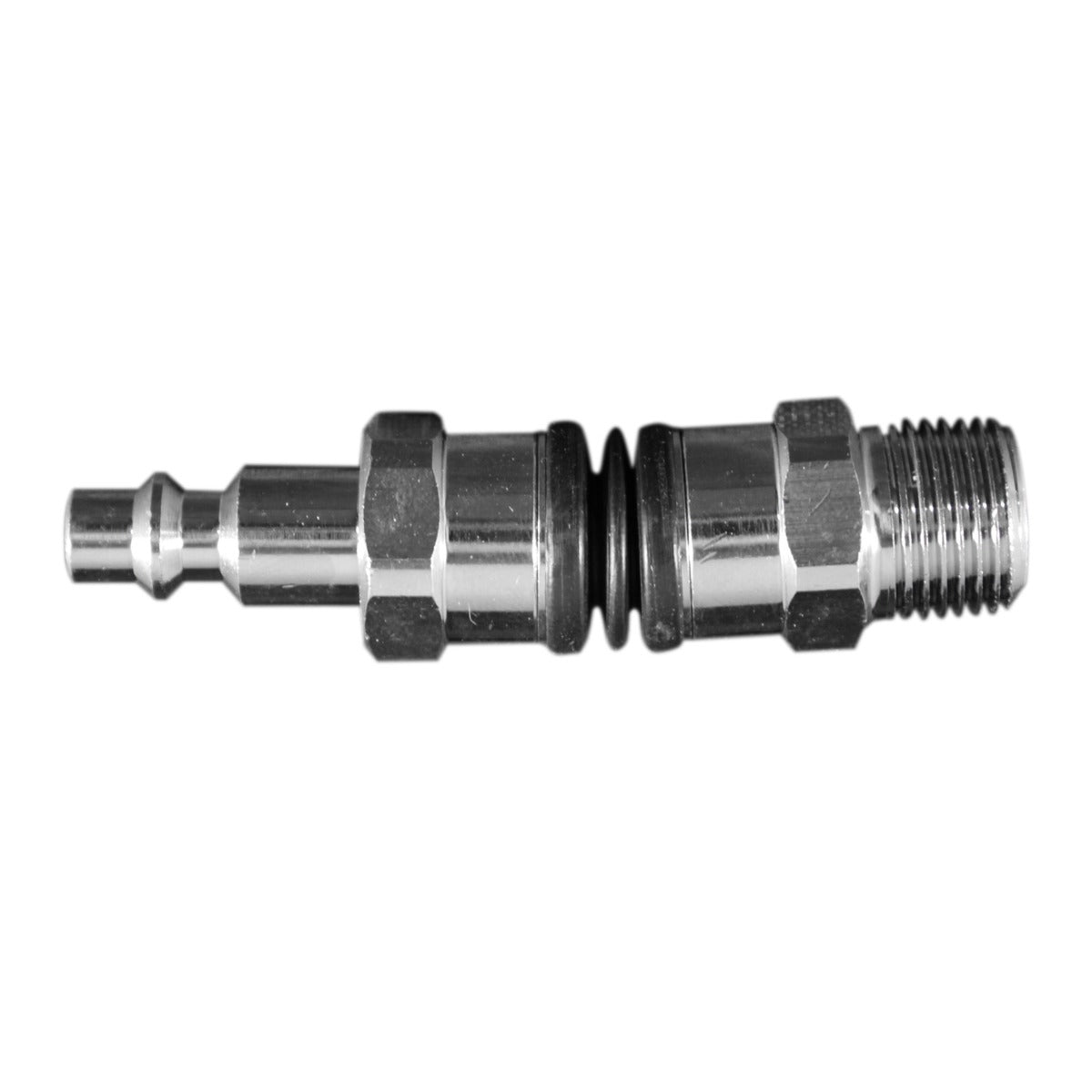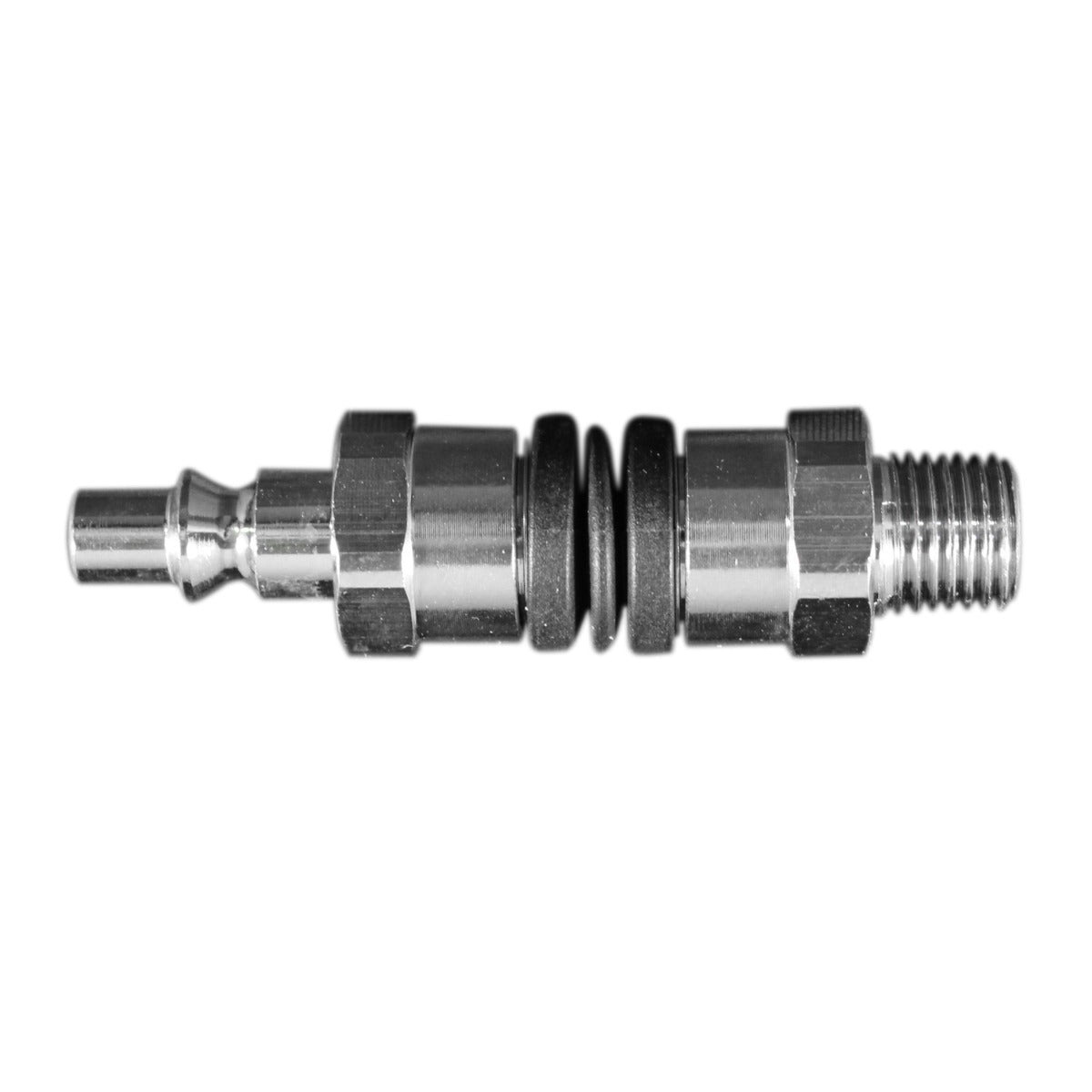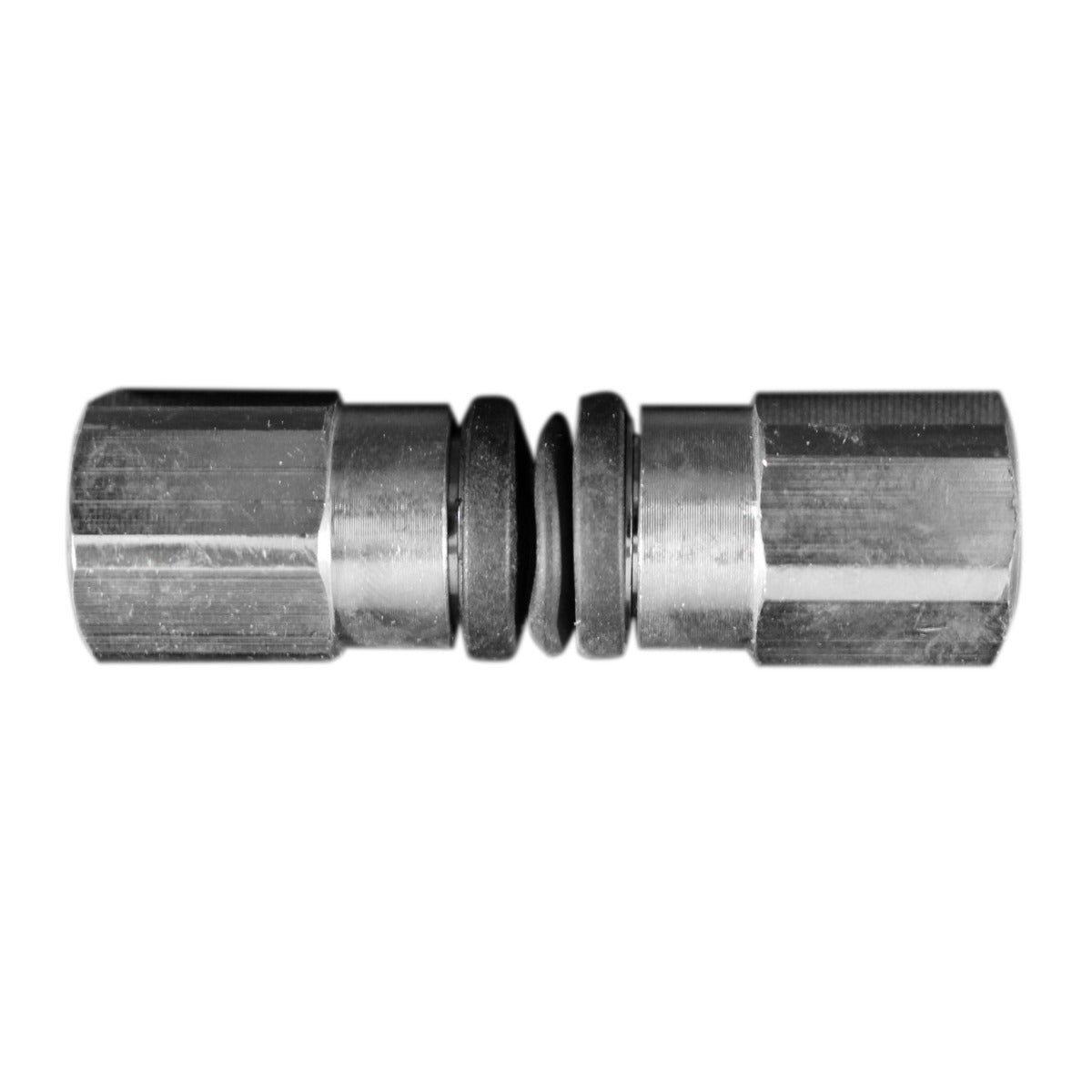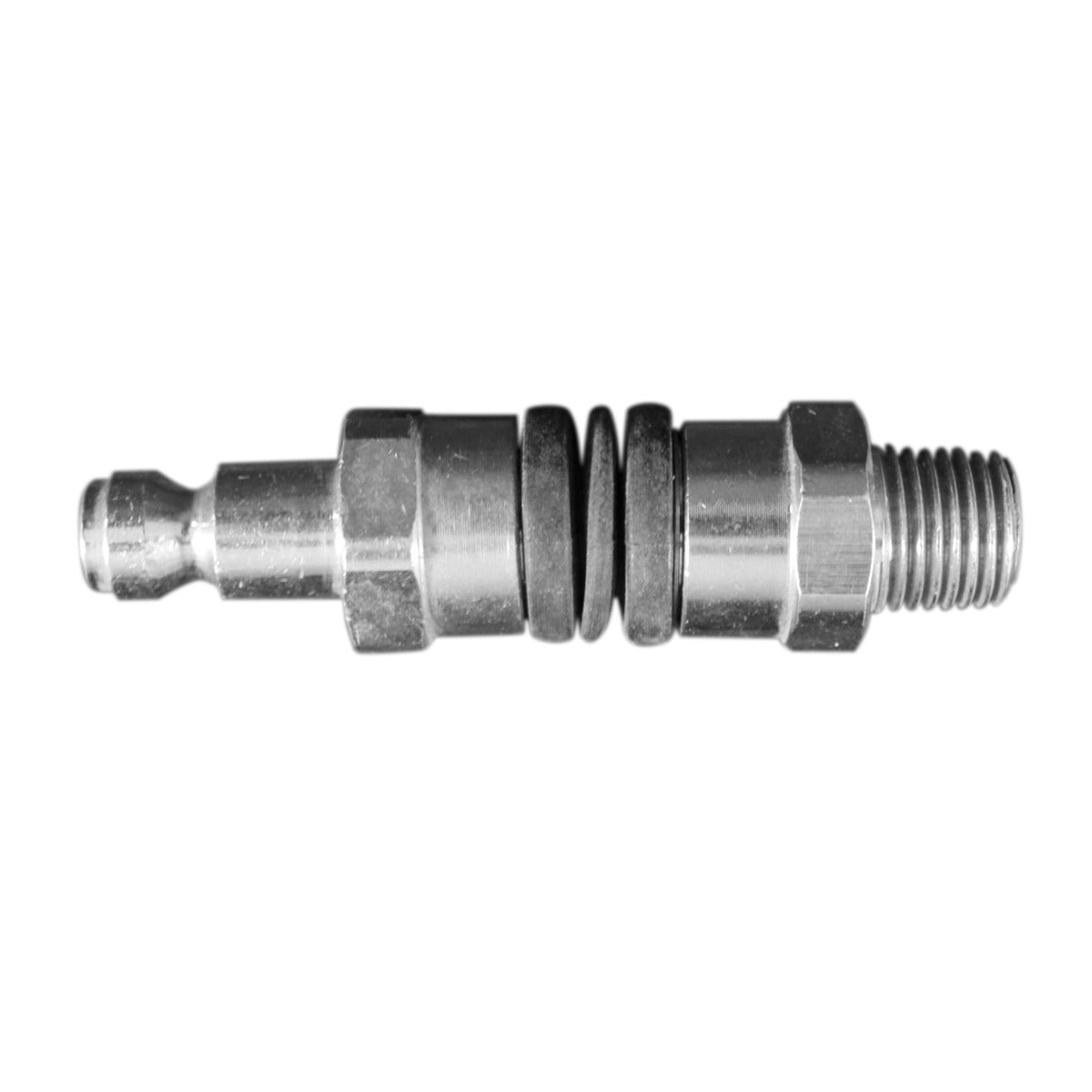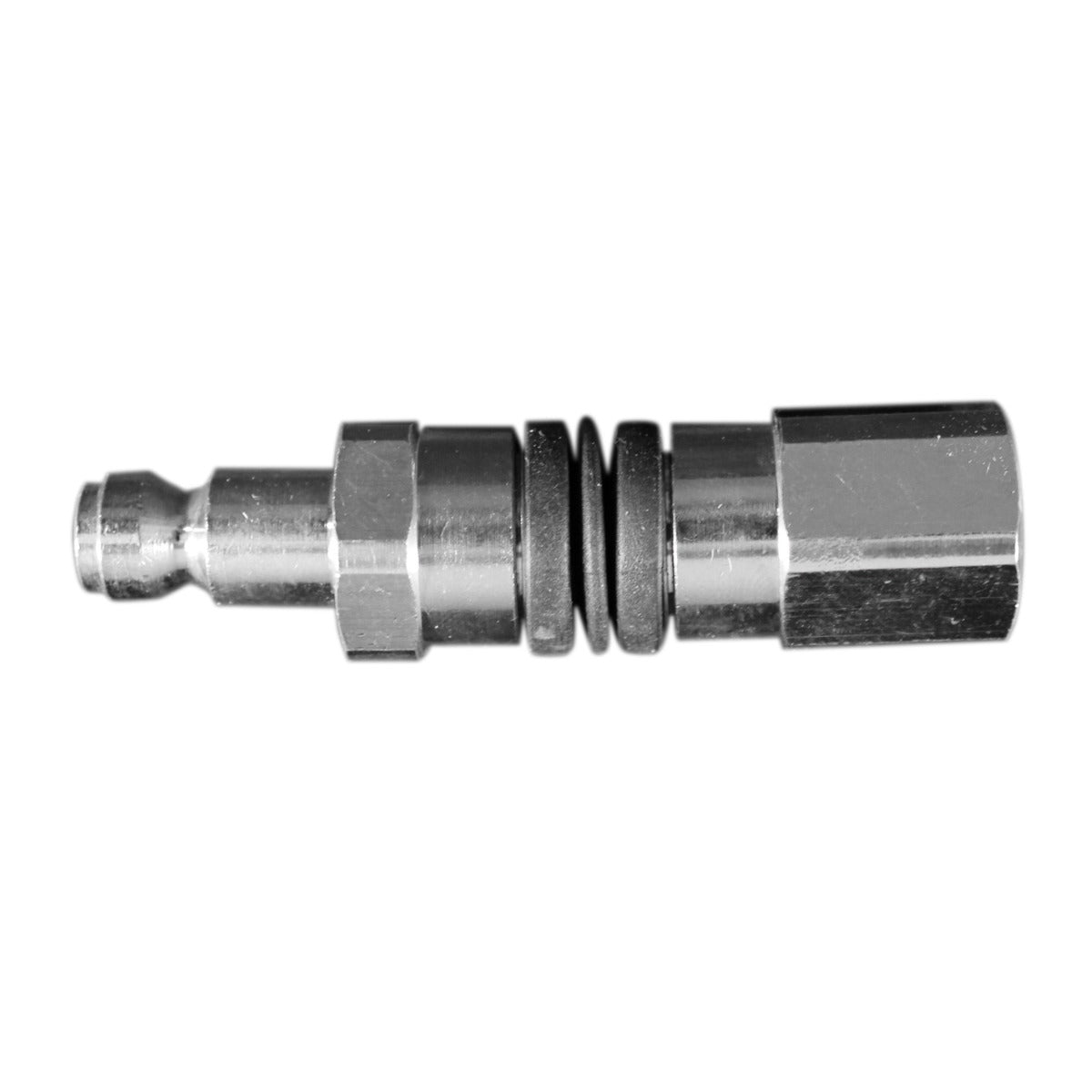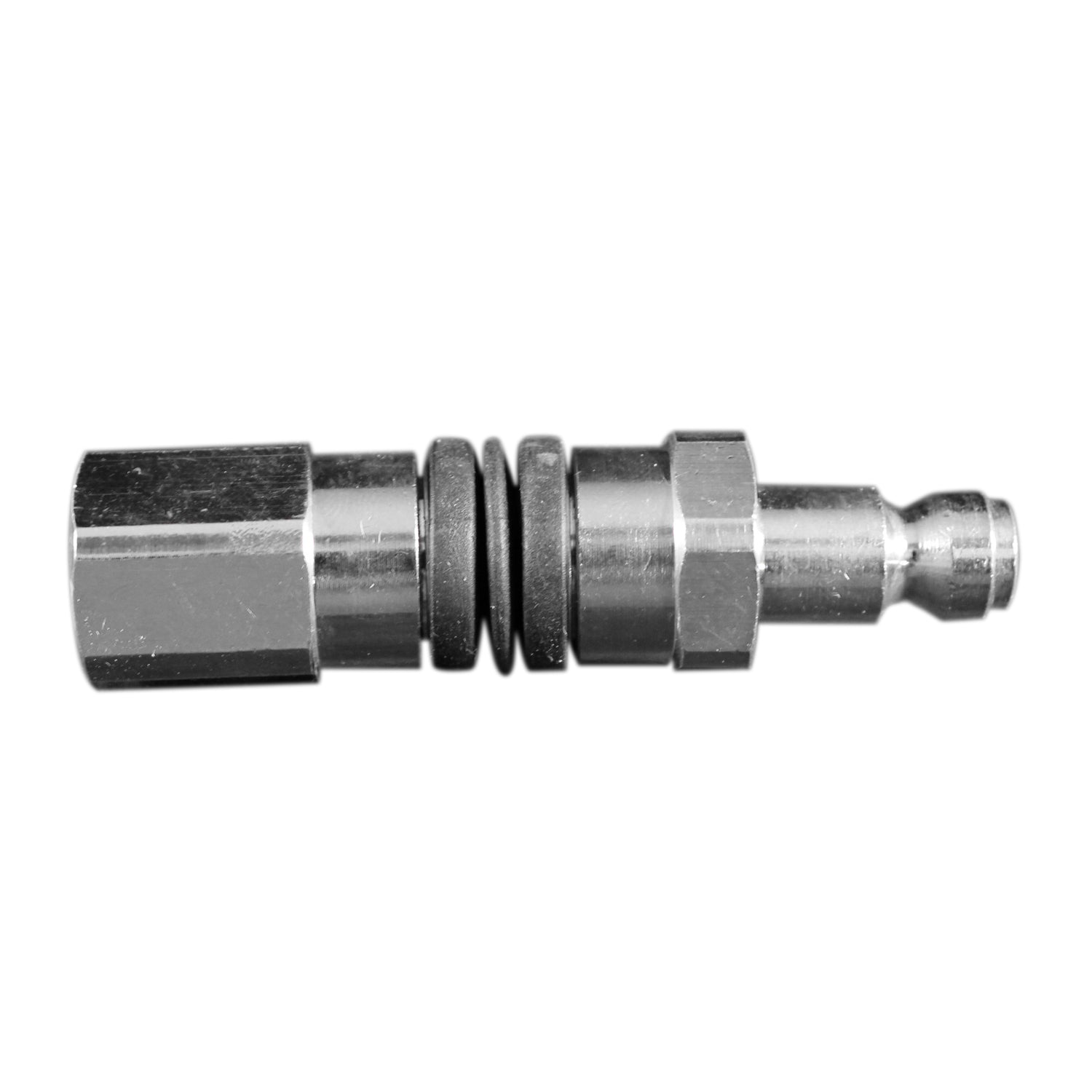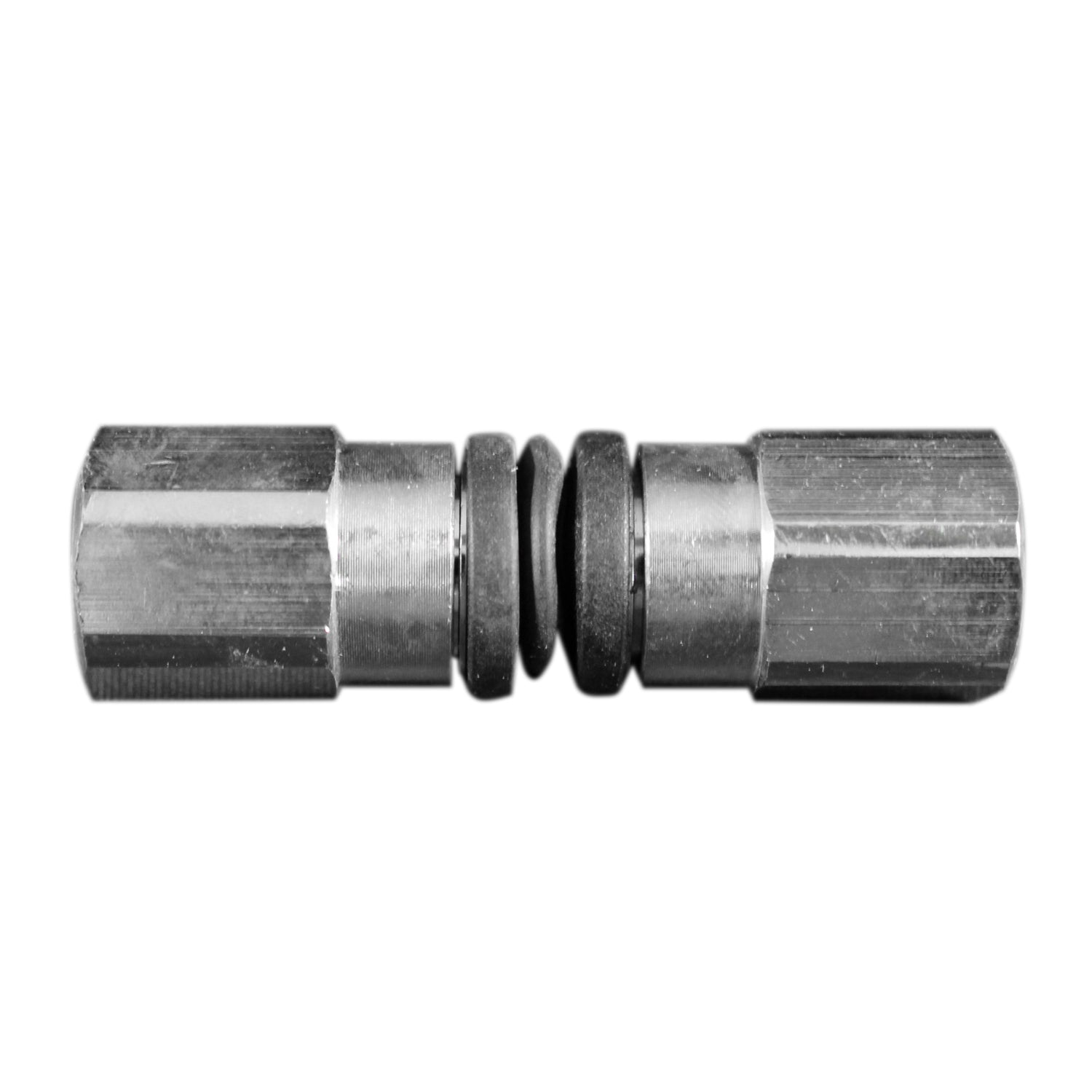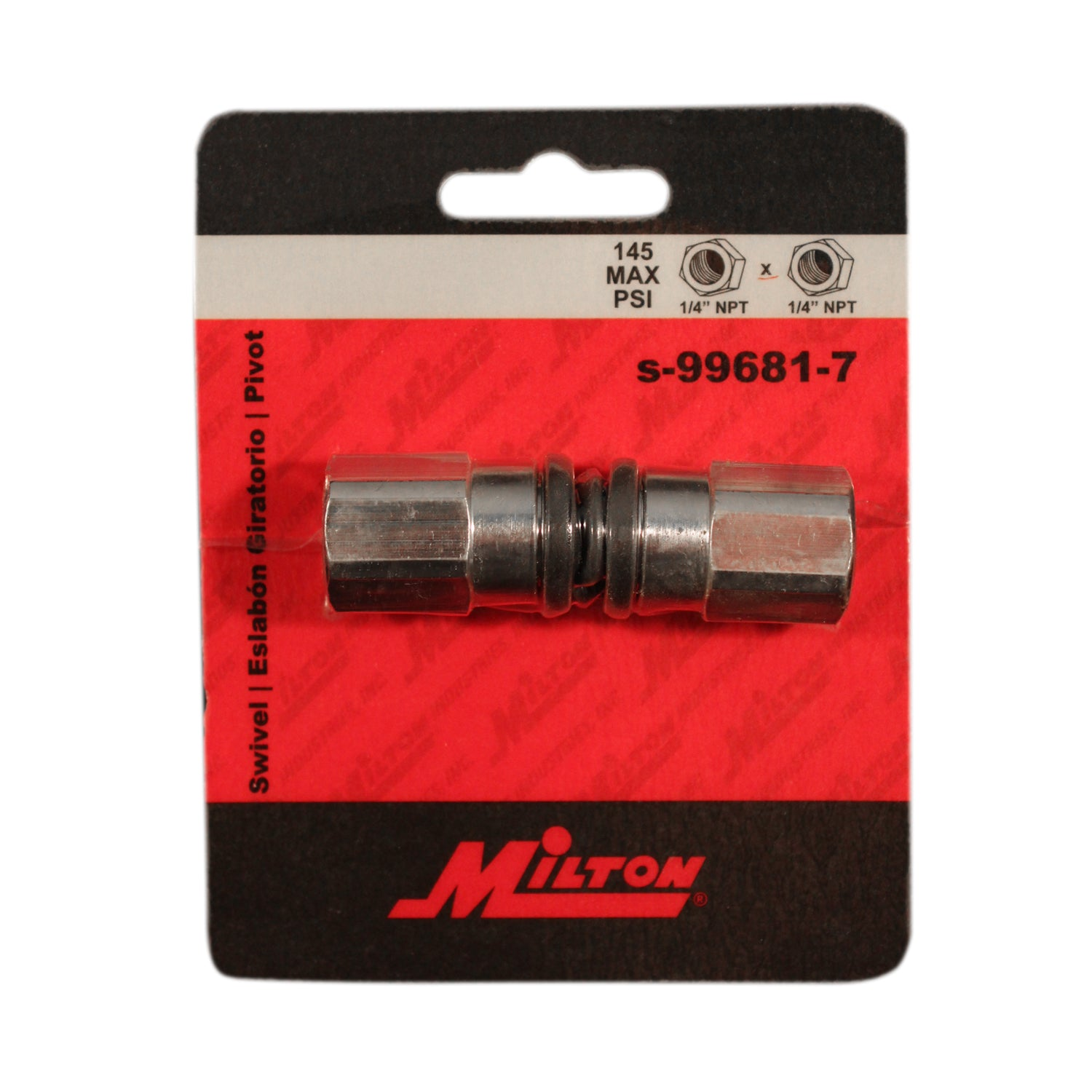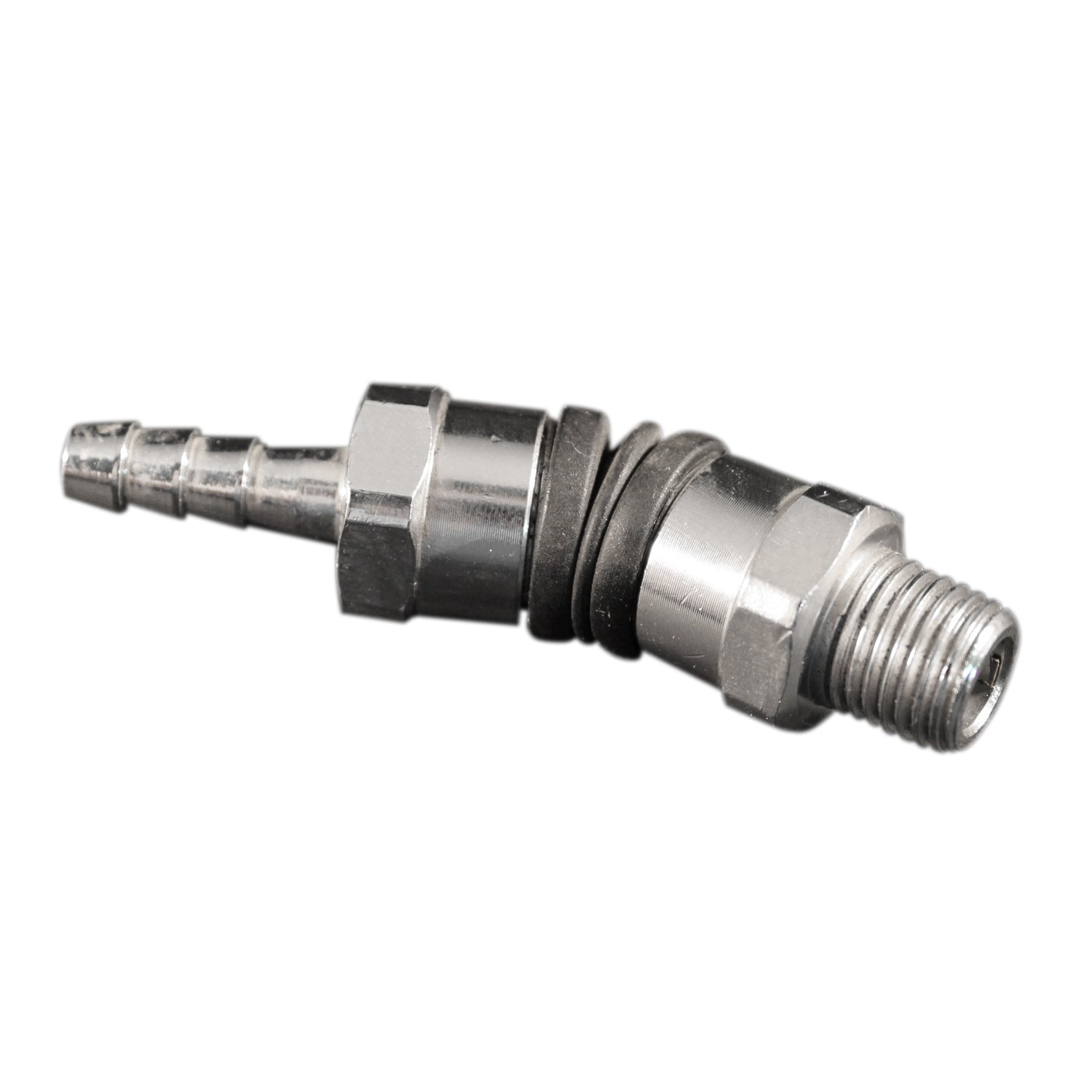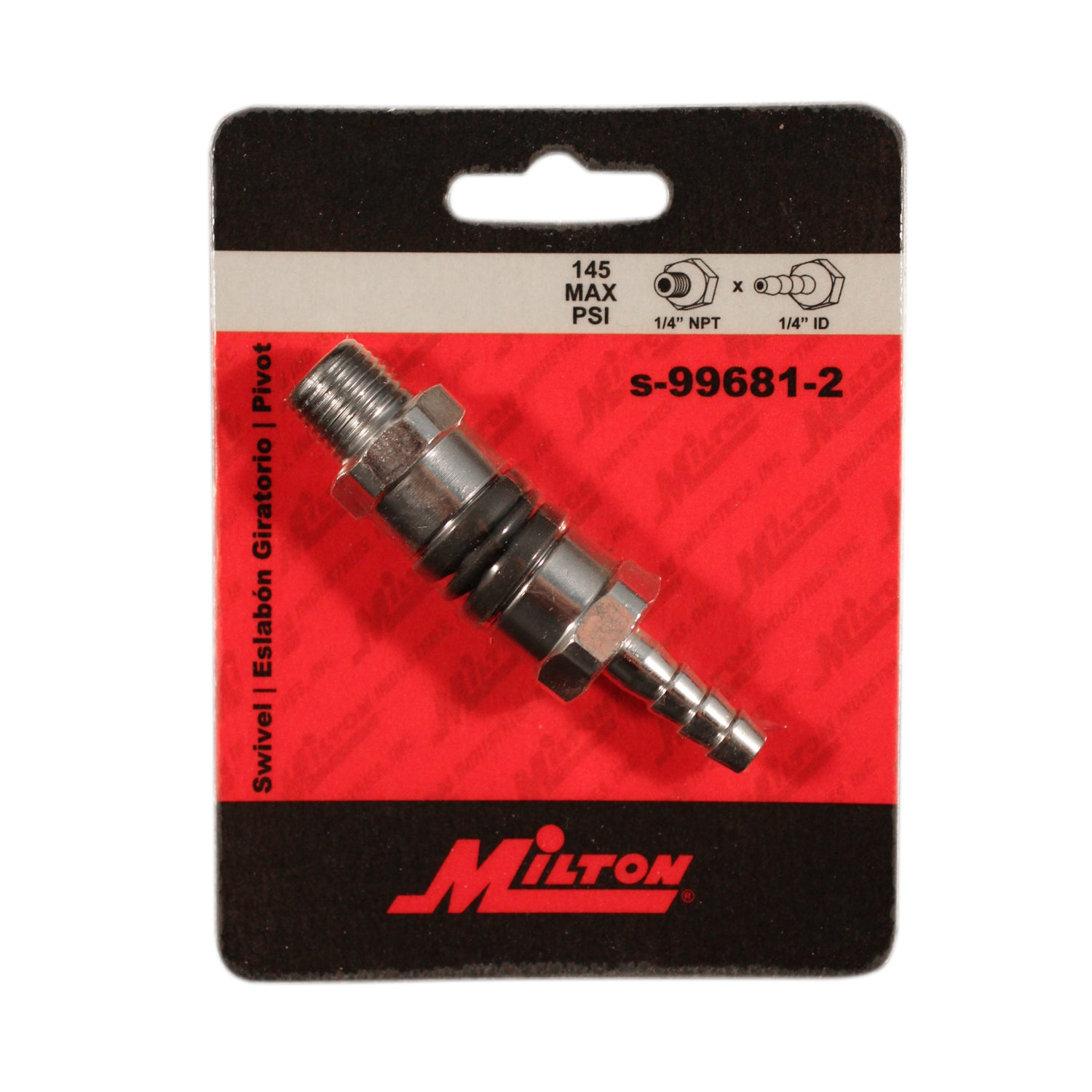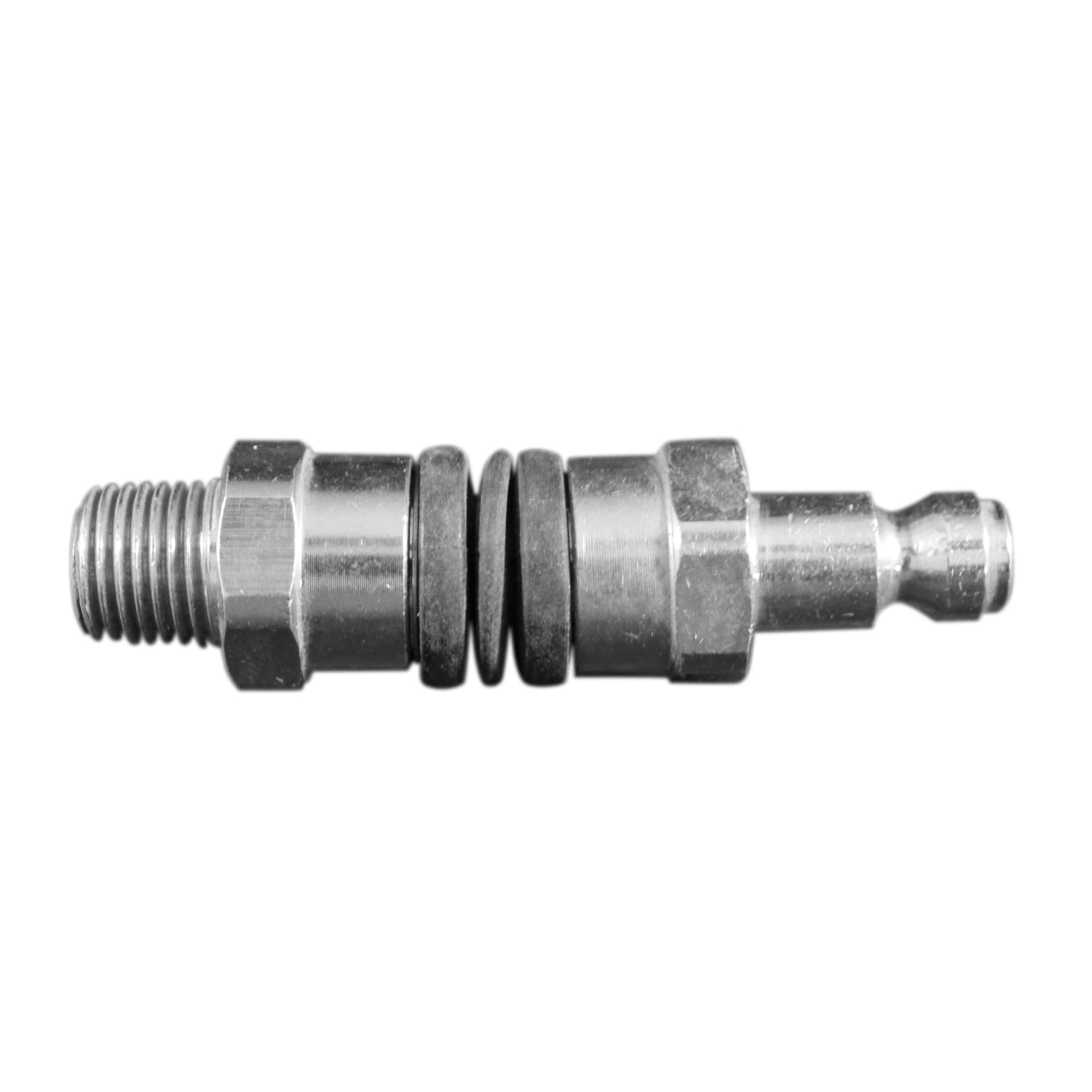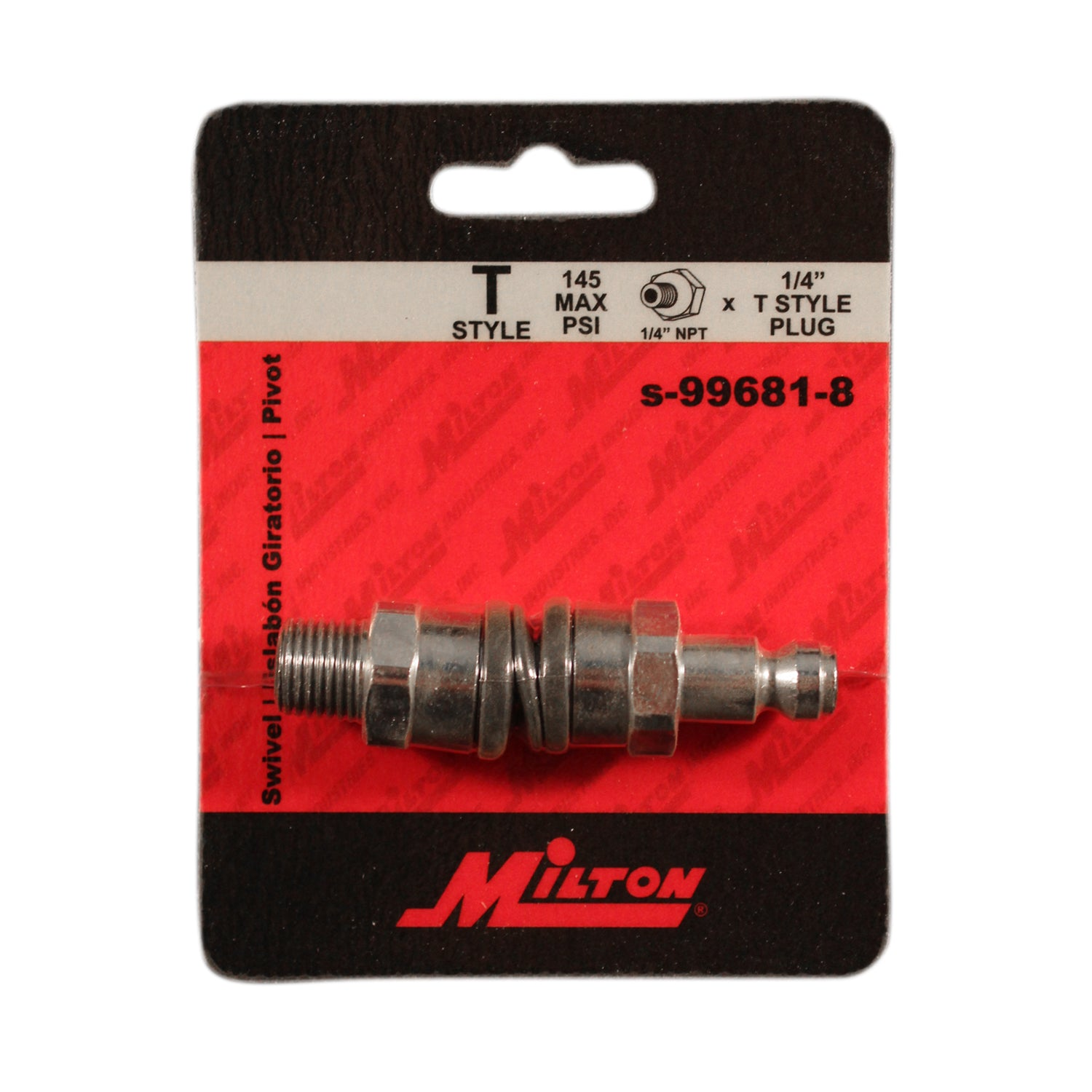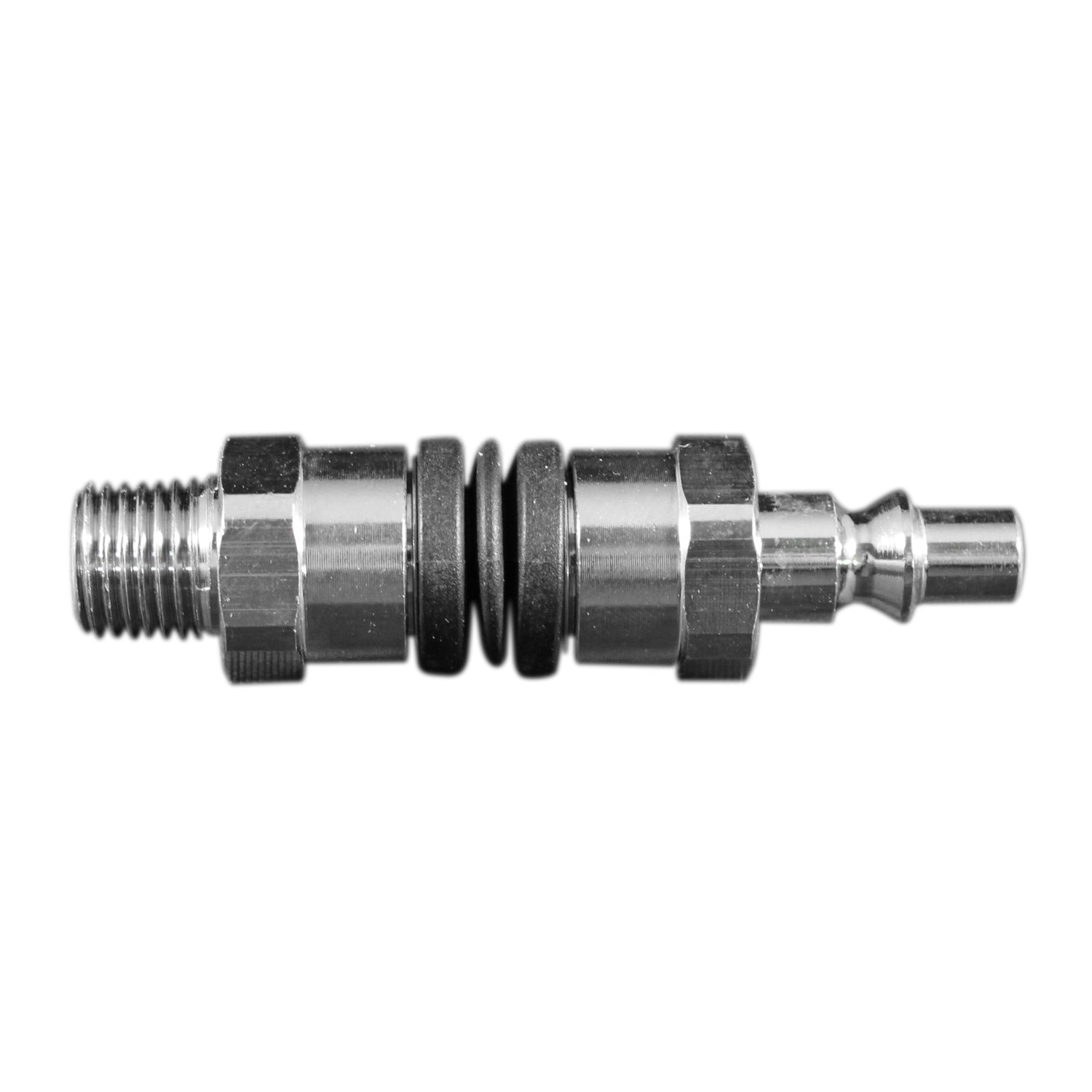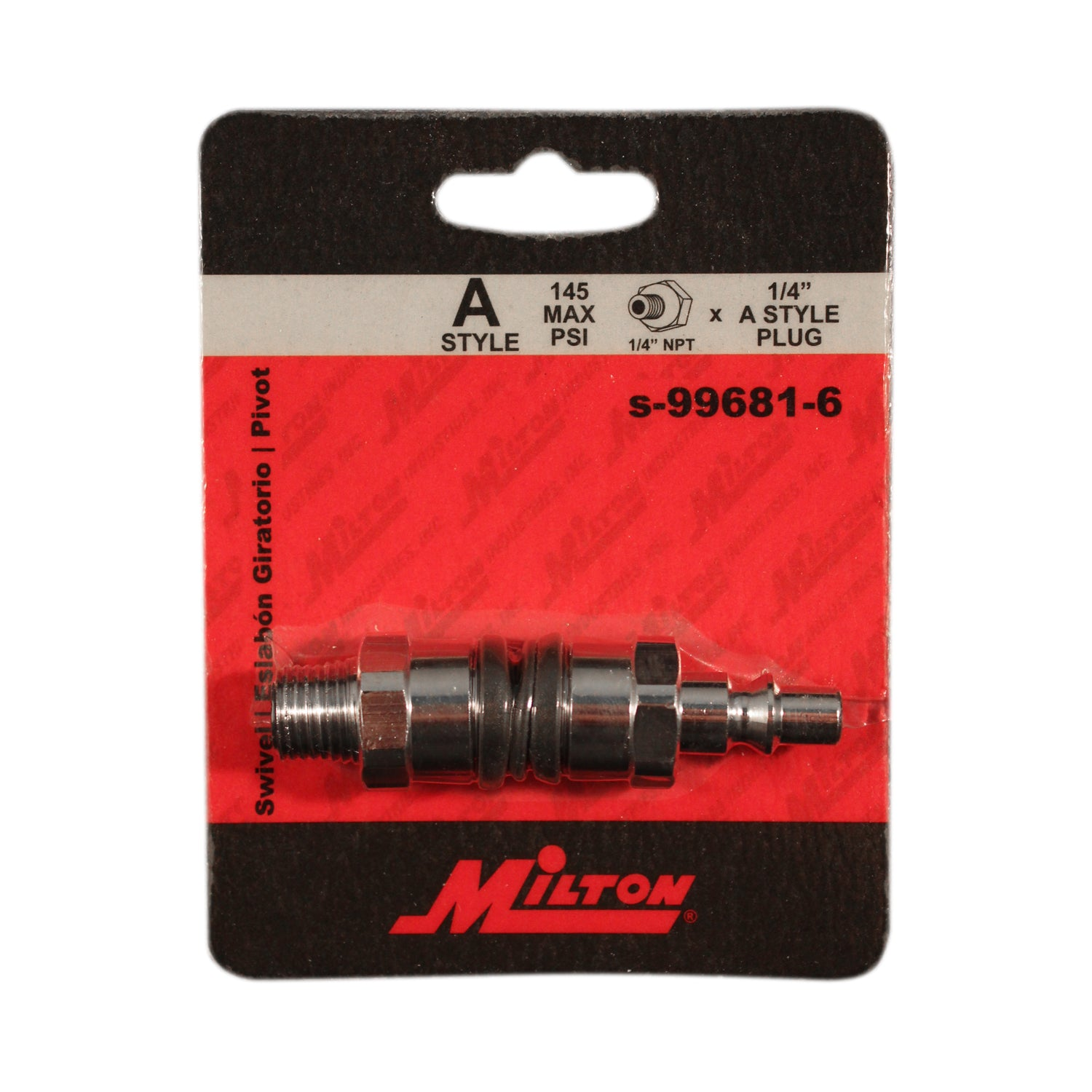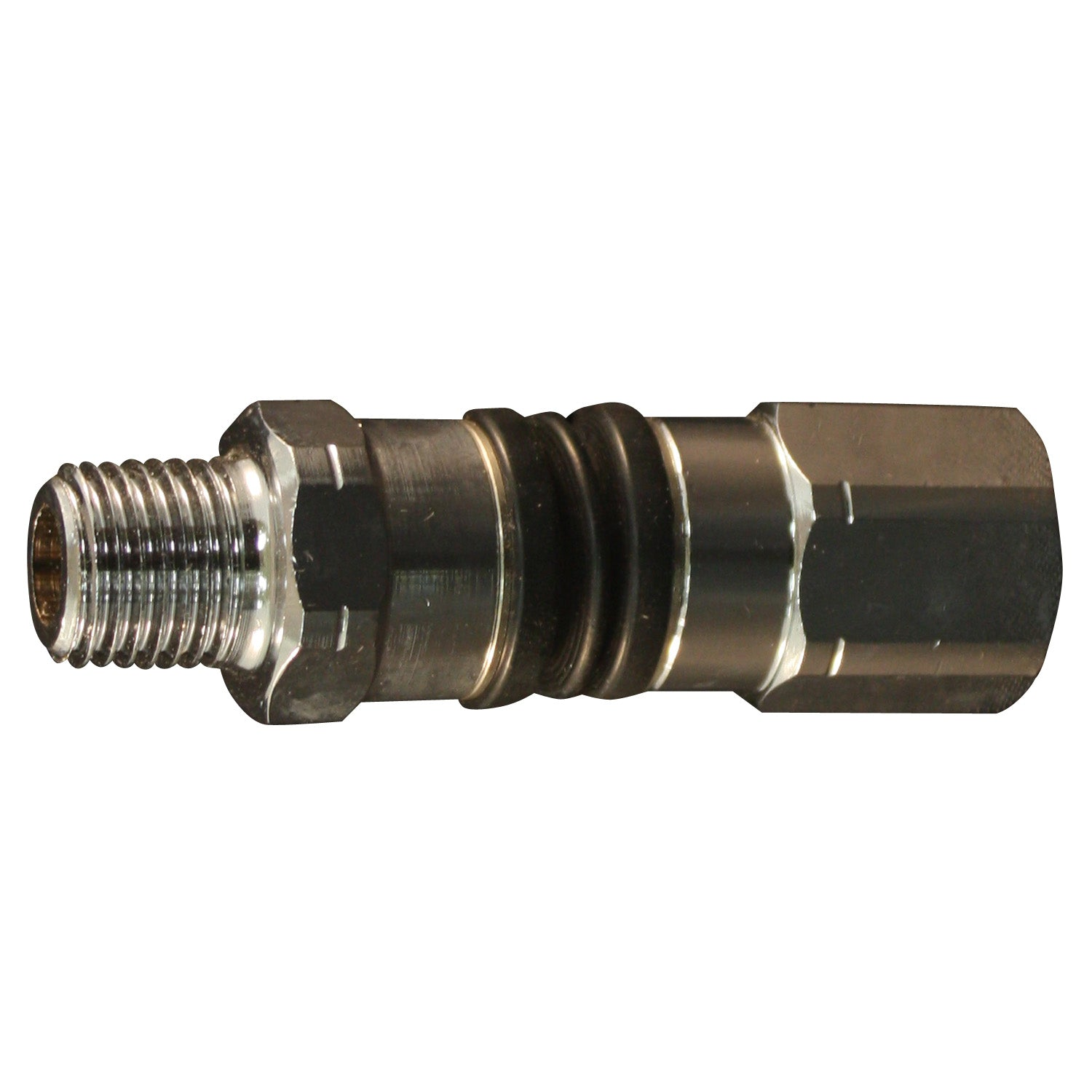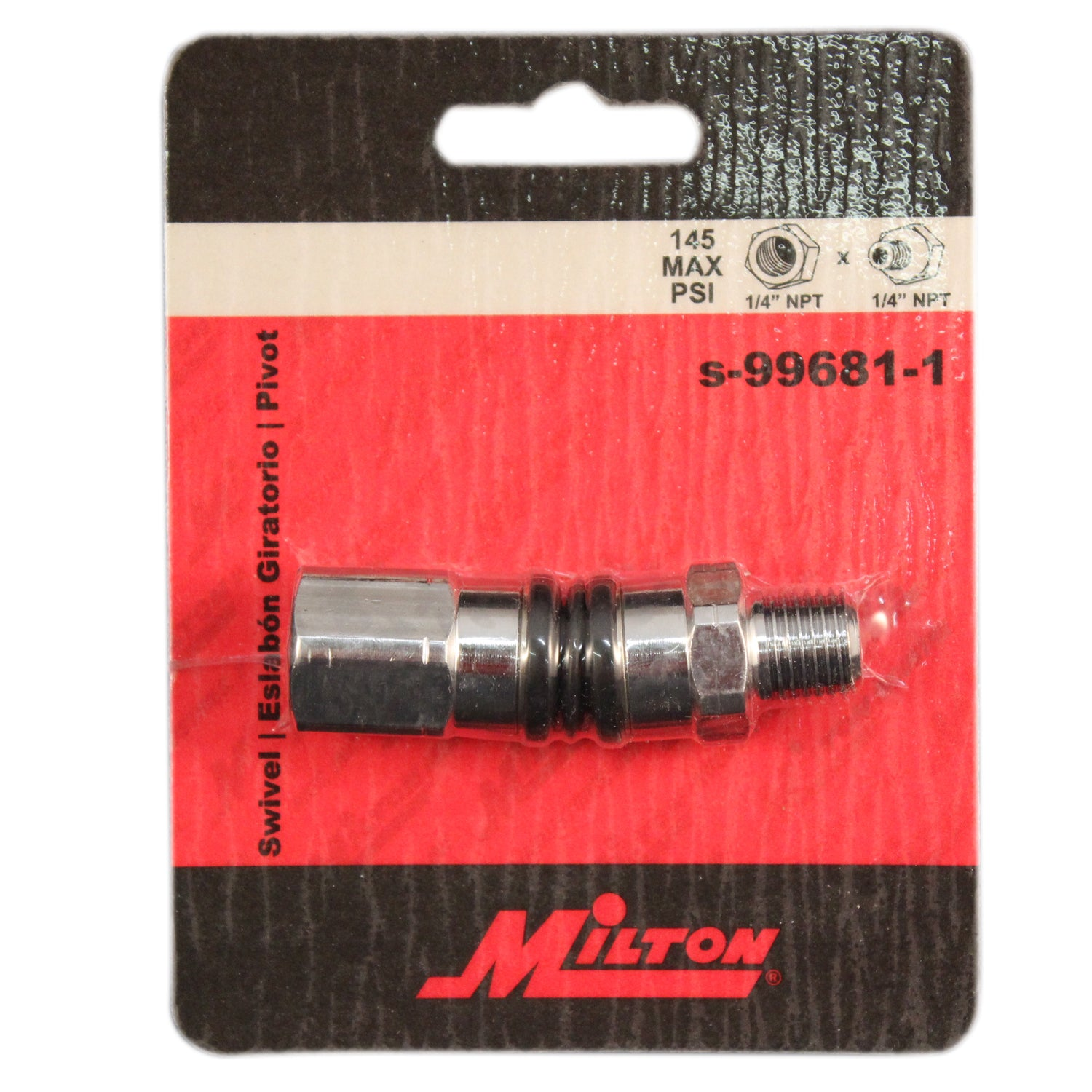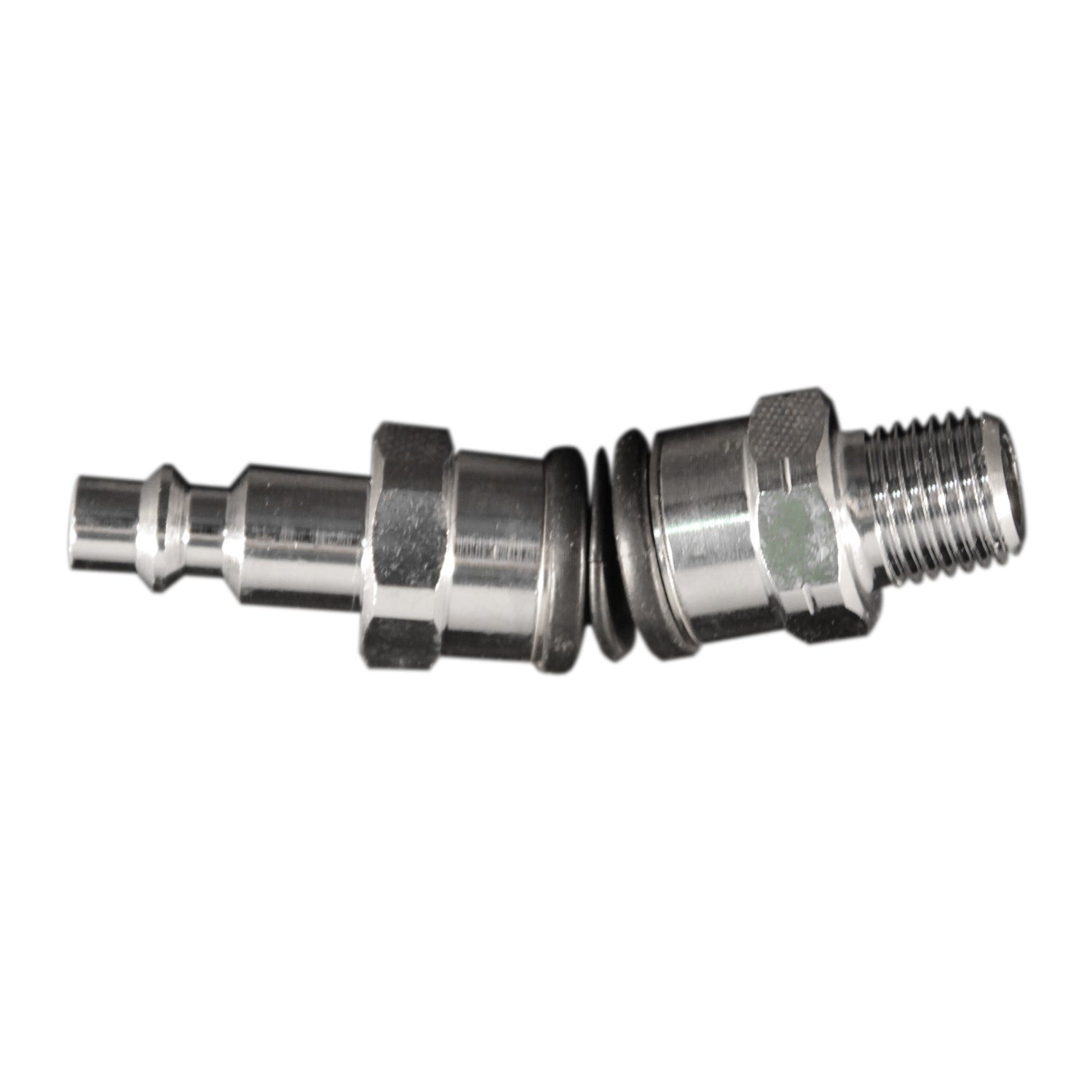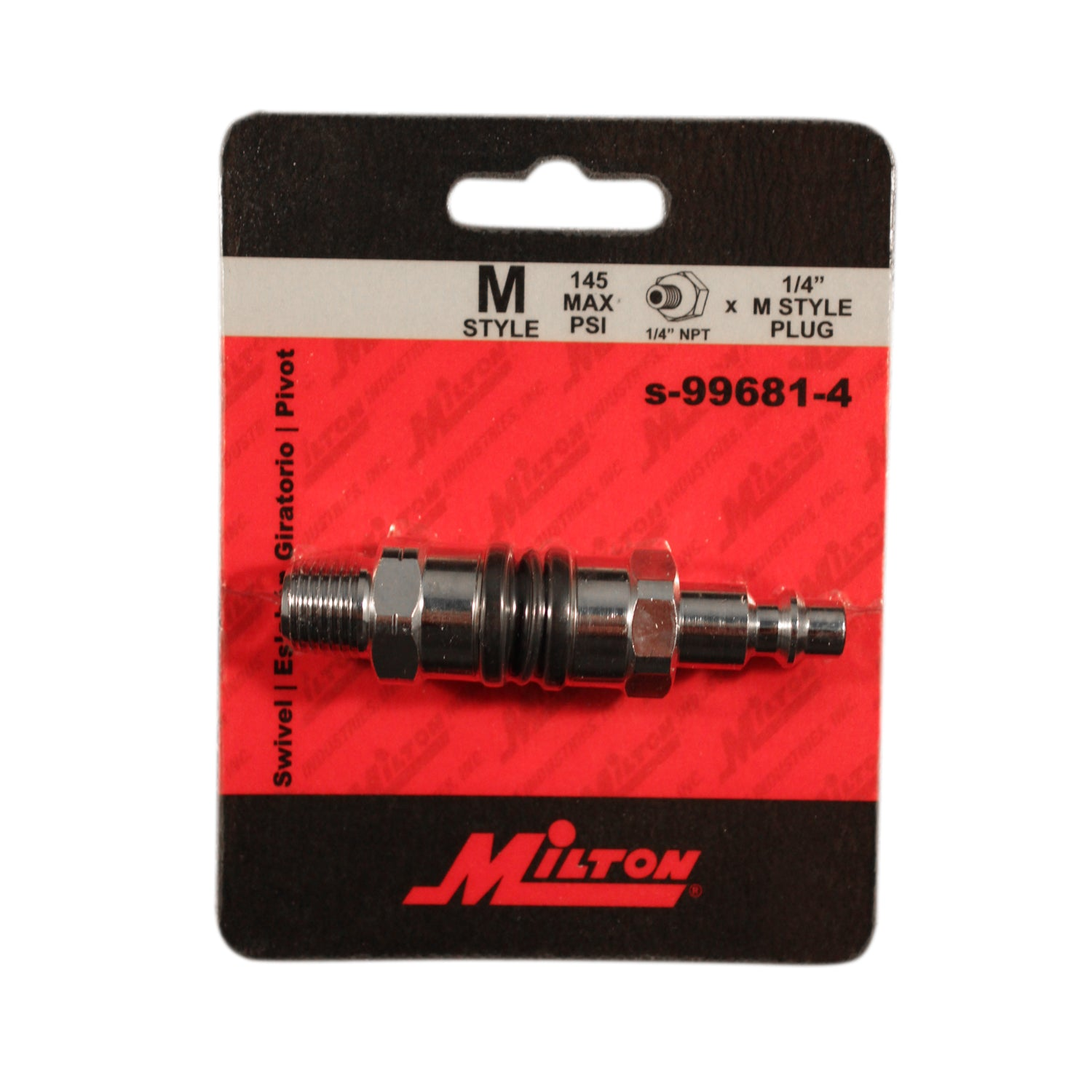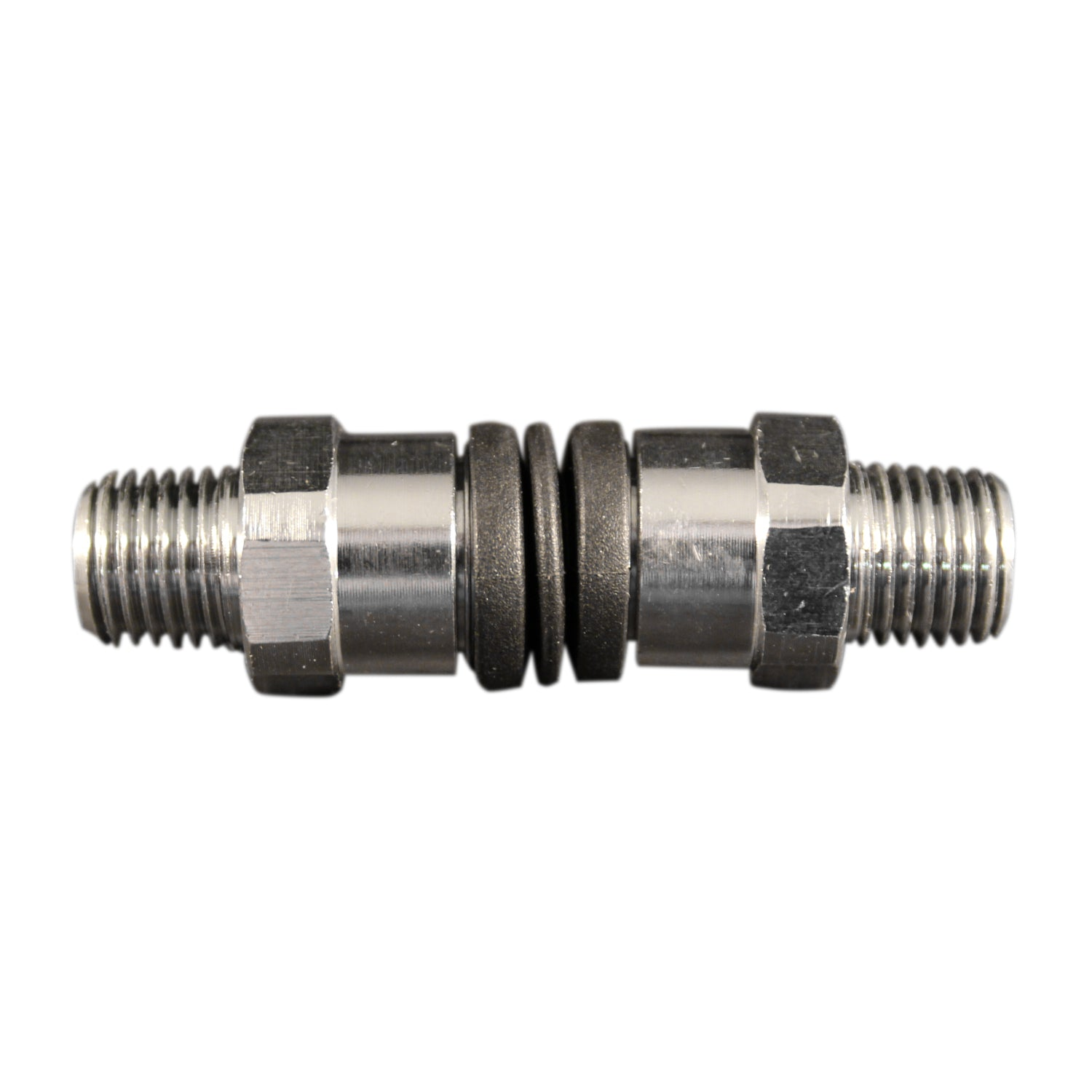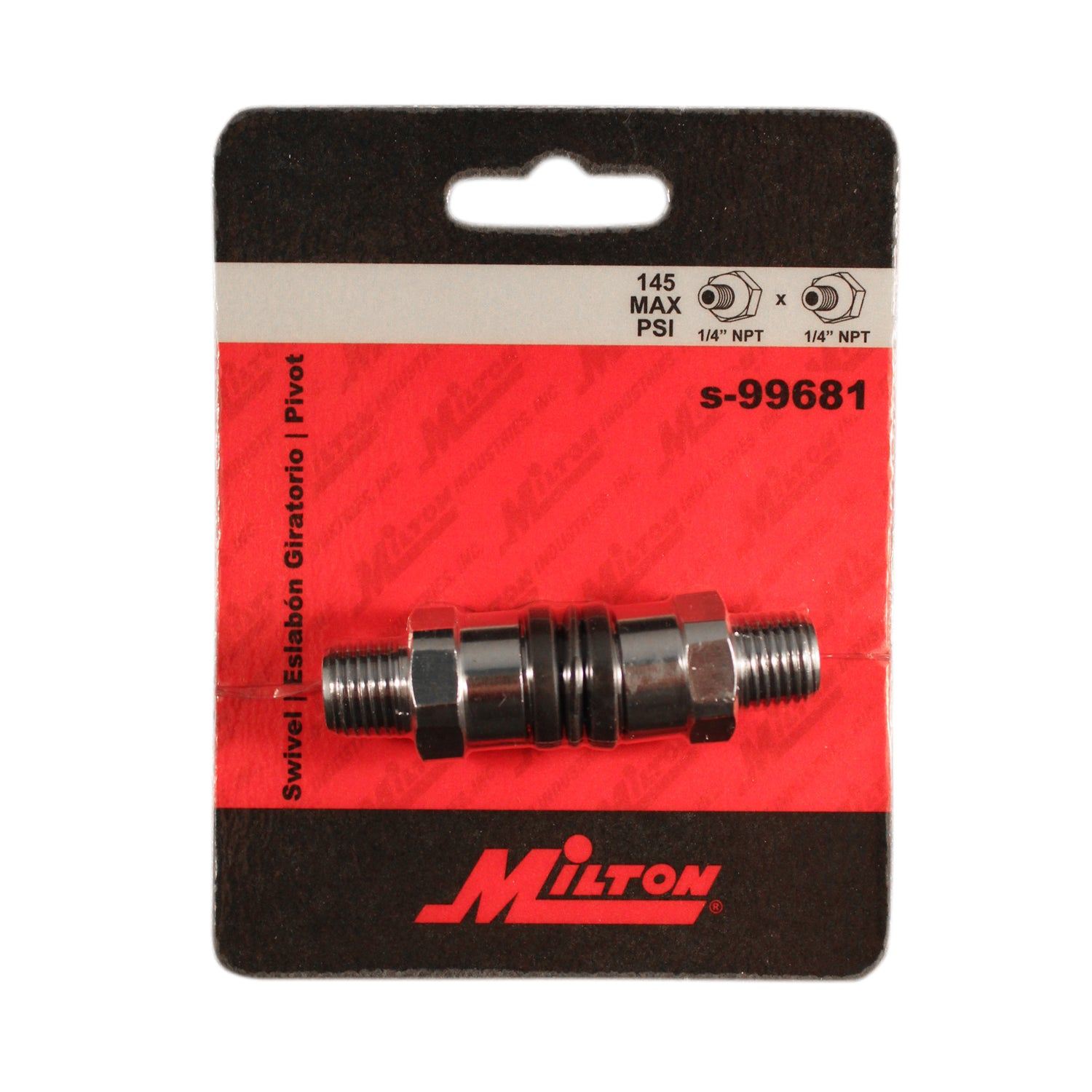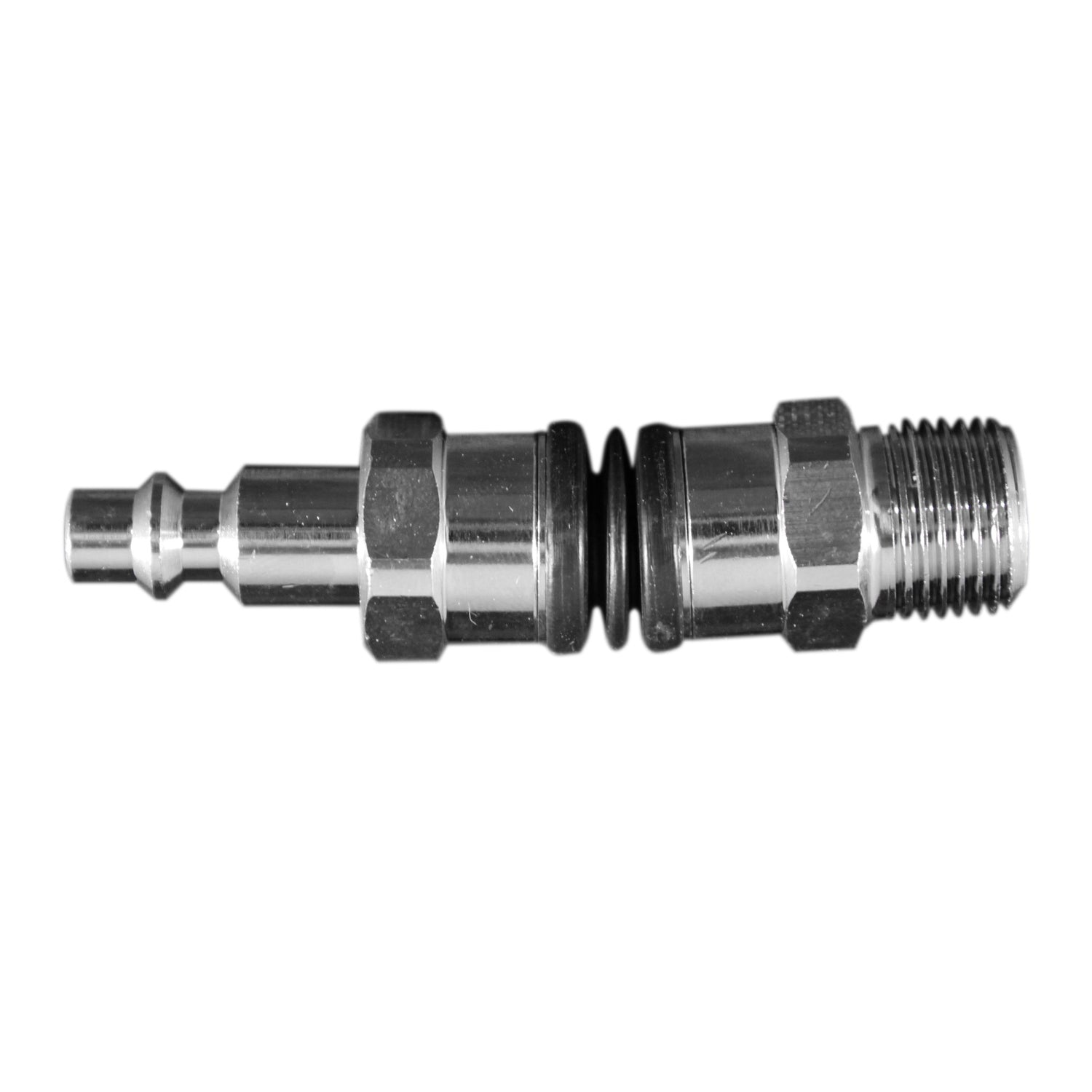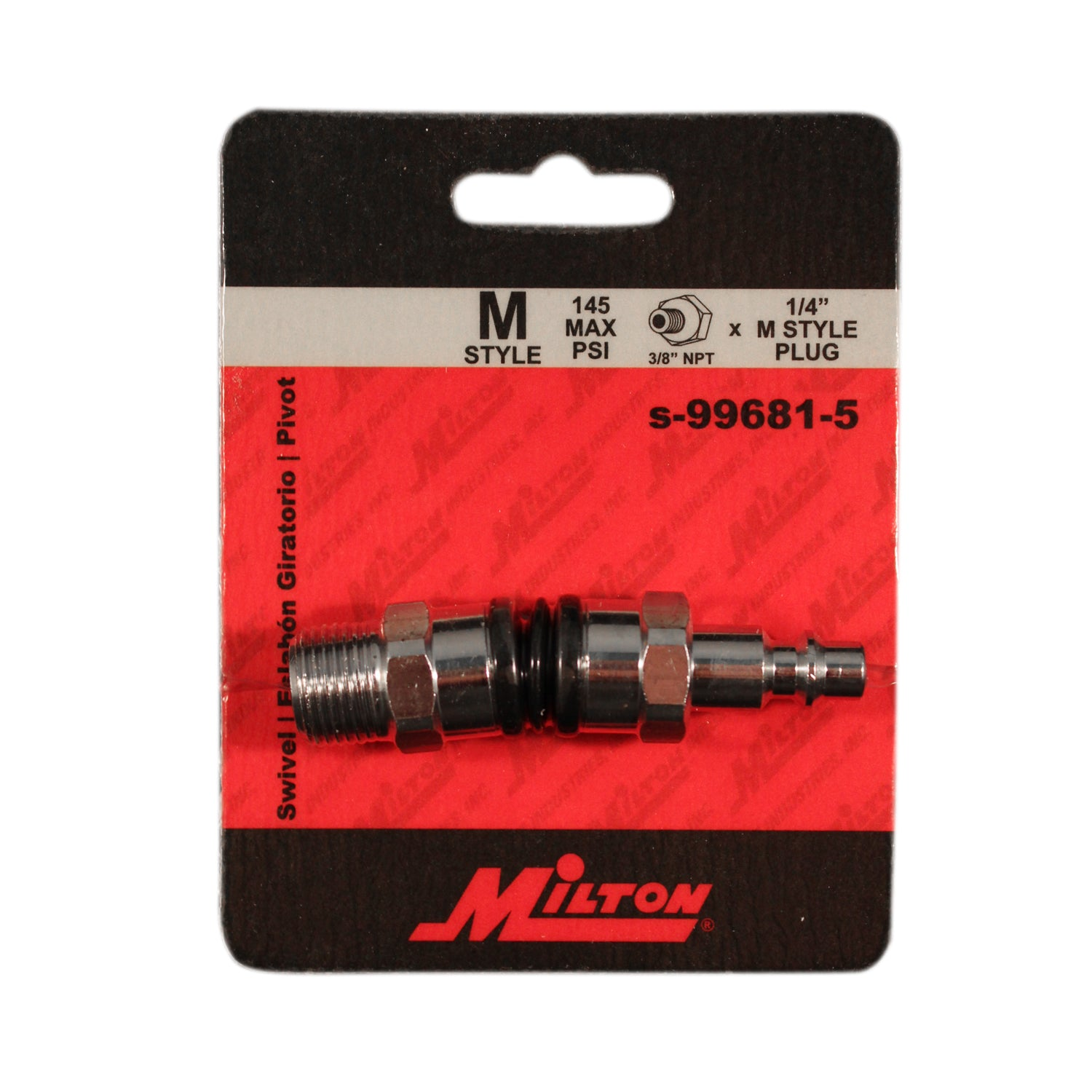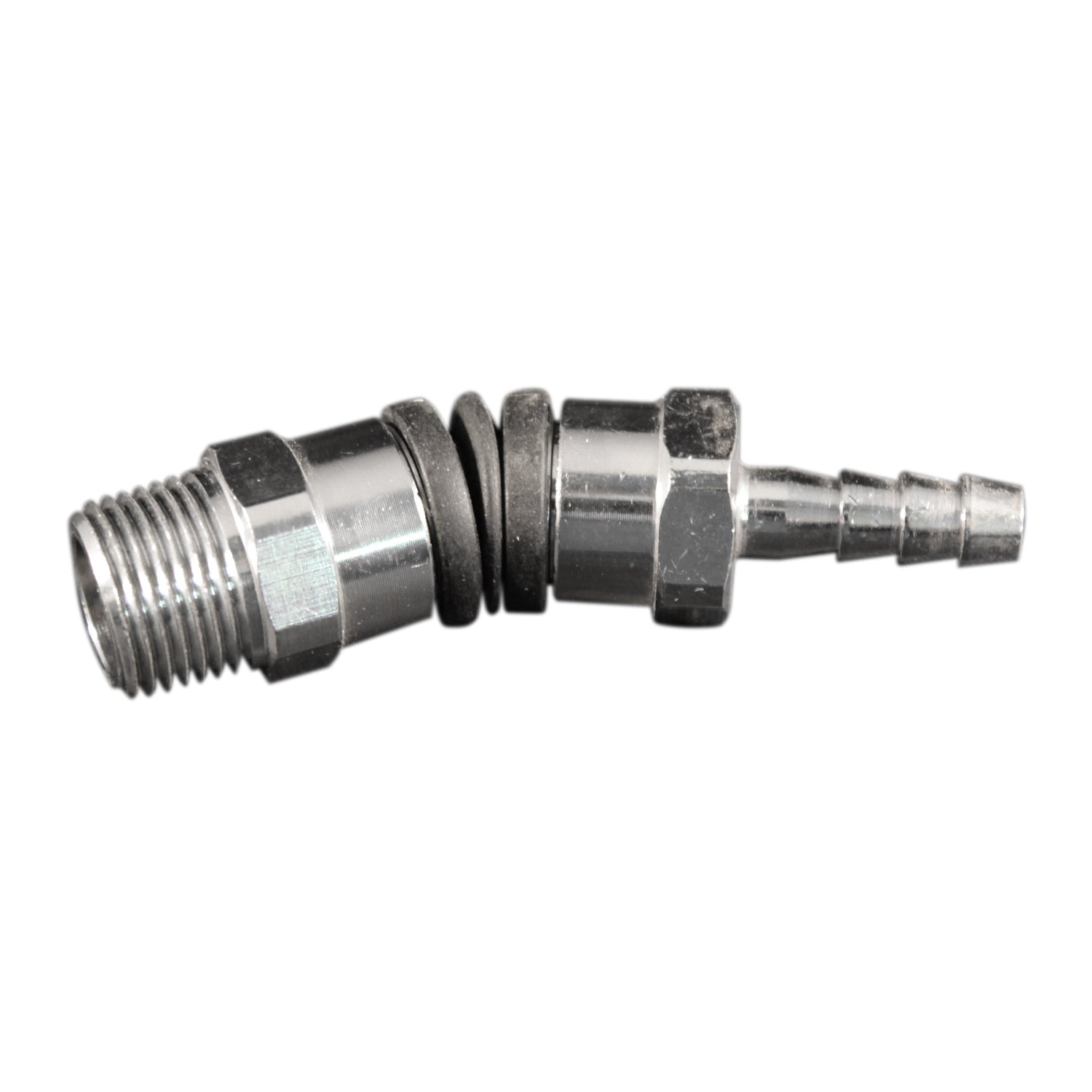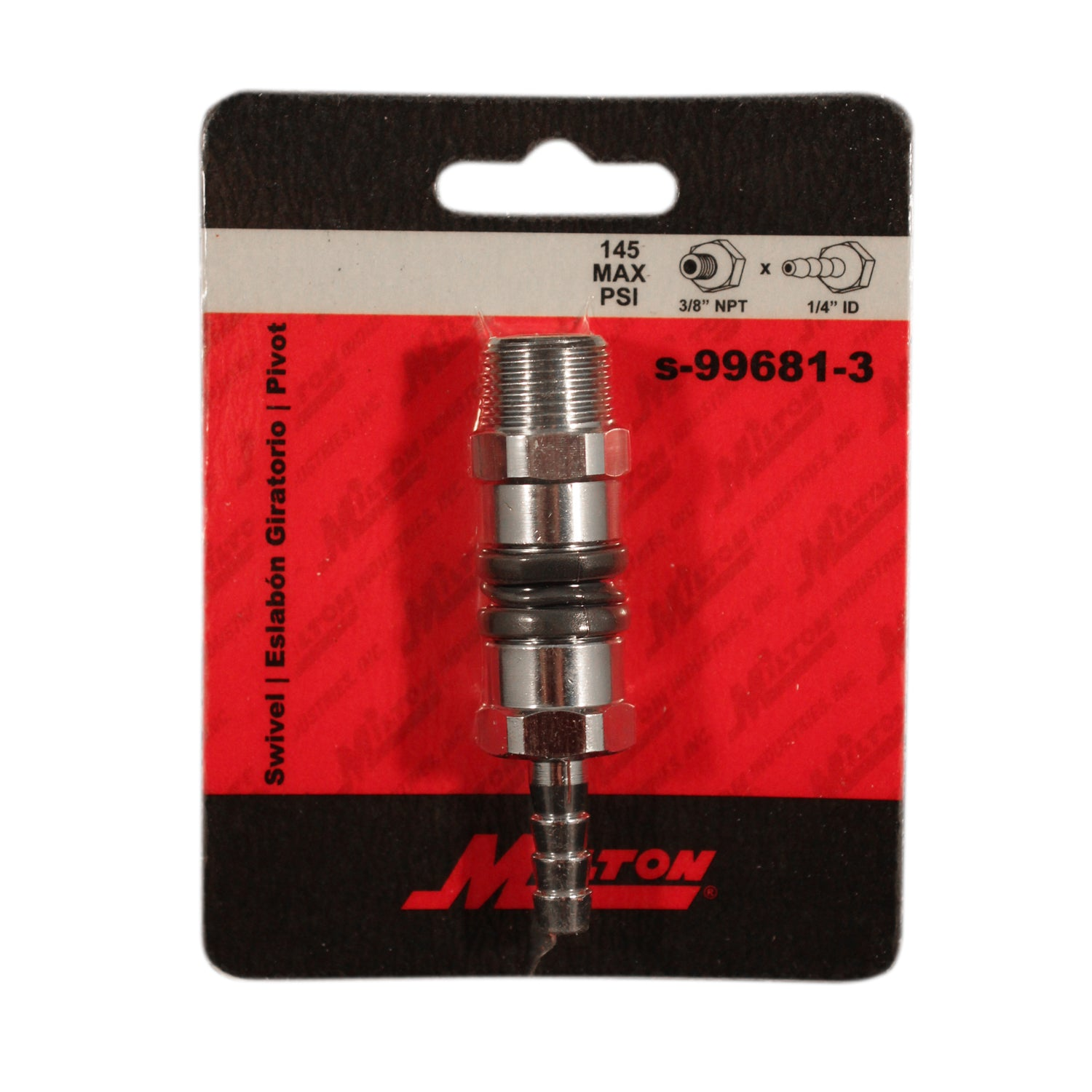Save: 58% 59% 66% 35%### Variable Angle Swivel Fitting

Milton Industries Inc.®

Part #: S-99681-9W
UPC Code: 30937312730
UPC Code: 30937312730
UPC Code: 30937312143
UPC Code: 30937312143
UPC Code: 30937312044
UPC Code: 30937312716
UPC Code: 30937312044
UPC Code: 30937312129
UPC Code: 30937312020
UPC Code: 30937312129
UPC Code: 30937312020
UPC Code: 30937312082
UPC Code: 30937312006
UPC Code: 30937312082
UPC Code: 30937312716
UPC Code: 30937312006
UPC Code: 30937312105
UPC Code: 30937312105
UPC Code: 30937312068
UPC Code: 30937312068
Number of Items: 1
Number of Items: 5
Number of Items: 1
Number of Items: 5
Number of Items: 1
Number of Items: 5
Number of Items: 5
Number of Items: 1
Number of Items: 1
Number of Items: 5
Number of Items: 5
Number of Items: 1
Number of Items: 1
Number of Items: 5
Number of Items: 1
Number of Items: 5
Number of Items: 1
Number of Items: 5
Number of Items: 1
Number of Items: 5
• Maximum working pressure of 145 pounds per square inch
• Temperature range of -37 degrees Fahrenheit to 176 degrees Fahrenheit
• Maximum working pressure of 145 pounds per square inch
• Temperature range of -37 degrees Fahrenheit to 176 degrees Fahrenheit
• Maximum working pressure of 145 pounds per square inch
• Temperature range of -37 degrees Fahrenheit to 176 degrees Fahrenheit
• Maximum working pressure of 145 pounds per square inch
• Temperature range of -37 degrees Fahrenheit to 176 degrees Fahrenheit
• Maximum working pressure of 145 pounds per square inch
• Temperature range of -37 degrees Fahrenheit to 176 degrees Fahrenheit
• Maximum working pressure of 145 pounds per square inch
• Temperature range of -37 degrees Fahrenheit to 176 degrees Fahrenheit
• Maximum working pressure of 145 pounds per square inch
• Temperature range of -37 degrees Fahrenheit to 176 degrees Fahrenheit
• Maximum working pressure of 145 pounds per square inch
• Temperature range of -37 degrees Fahrenheit to 176 degrees Fahrenheit
• Maximum working pressure of 145 pounds per square inch
• Temperature range of -37 degrees Fahrenheit to 176 degrees Fahrenheit
• Maximum working pressure of 145 pounds per square inch
• Temperature range of -37 degrees Fahrenheit to 176 degrees Fahrenheit
• Maximum working pressure of 145 pounds per square inch
• Temperature range of -37 degrees Fahrenheit to 176 degrees Fahrenheit
• Maximum working pressure of 145 pounds per square inch
• Temperature range of -37 degrees Fahrenheit to 176 degrees Fahrenheit
• Maximum working pressure of 145 pounds per square inch
• Temperature range of -37 degrees Fahrenheit to 176 degrees Fahrenheit
• Maximum working pressure of 145 pounds per square inch
• Temperature range of -37 degrees Fahrenheit to 176 degrees Fahrenheit
• Maximum working pressure of 145 pounds per square inch
• Temperature range of -37 degrees Fahrenheit to 176 degrees Fahrenheit
• Maximum working pressure of 145 pounds per square inch
• Temperature range of -37 degrees Fahrenheit to 176 degrees Fahrenheit
• Maximum working pressure of 145 pounds per square inch
• Temperature range of -37 degrees Fahrenheit to 176 degrees Fahrenheit
• Maximum working pressure of 145 pounds per square inch
• Temperature range of -37 degrees Fahrenheit to 176 degrees Fahrenheit
• Maximum working pressure of 145 pounds per square inch
• Temperature range of -37 degrees Fahrenheit to 176 degrees Fahrenheit
• Maximum working pressure of 145 pounds per square inch
• Temperature range of -37 degrees Fahrenheit to 176 degrees Fahrenheit
\$12.22
\$0.00
Save: 58% 59% 66% 35%

### Quantity### Variable Angle Swivel Fitting

Milton® Variable angle swivel fittings rotate 360 degrees at a 45 degree angle in any direction, virtually eliminating hose twisting and kinking. Rubber dust cover keeps dirt and moisture out.

Milton® Variable angle swivel fittings rotate 360 degrees at a 45 degree angle in any direction, virtually eliminating hose twisting and kinking. Rubber dust cover keeps dirt and moisture out.

 Type of Hose : Type of fiiting : Thread Size : SCFM : PSI range :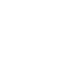### FREE SHIPPING

When you spend \$75+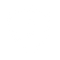### WARRANTY INFO

Offering a range of policies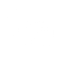### NEED HELP

Contact our support team today### FIND A DISTRIBUTOR

Locate the closest inventory